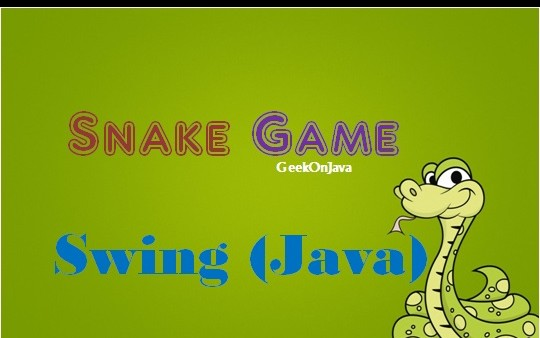{{announcement.body}}
{{announcement.title}}

# How to Make a Snake Game in Swing

DZone 's Guide to

# How to Make a Snake Game in Swing

### Learn to create a full Snake game in Swing using width and height constraints and the ImageIcon class.

· Java Zone ·
Free Resource

Comment (0)

Save
{{ articles.views | formatCount}} Views

First we will define the constants used in our game.

``````private final int B_WIDTH = 300;
private final int B_HEIGHT = 300;
private final int DOT_SIZE = 10;
private final int ALL_DOTS = 900;
private final int RAND_POS = 29;
private final int DELAY = 140;``````

The B_WIDTH and B_HEIGHT constants determine the size of the board. The DOT_SIZE is the size of the apple and the dot of the snake.The ALL_DOTS constant defines the maximum number of possible dots on the board (900 = (300*300)/(10*10)). The RAND_POS constant is used to calculate a random position for an apple. The DELAY constant determines the speed of the game.

``````private final int x[] = new int[ALL_DOTS];
private final int y[] = new int[ALL_DOTS];``````

These two arrays store the x and y coordinates of all joints of a snake.

``````private void loadImages() {

ImageIcon iid = new ImageIcon("dot.png");
ball = iid.getImage();

ImageIcon iia = new ImageIcon("apple.png");
apple = iia.getImage();

}``````

In the loadImages() method we get the images for the game. The ImageIcon class is used for displaying PNG images.

``````private void initGame() {

dots = 3;

for (int z = 0; z < dots; z++) {
x[z] = 50 - z * 10;
y[z] = 50;
}

locateApple();

timer = new Timer(DELAY, this);
timer.start();
}``````

In the initGame() method we create the snake, randomly locate an apple on the board, and start the timer.

``````private void checkApple() {

if ((x == apple_x) && (y == apple_y)) {

dots++;
locateApple();
}
}``````

If the apple collides with the head, we increase the number of joints of the snake. We call the locateApple() method which randomly positions a new apple object.

In the move() method we have the key algorithm of the game. To understand it, look at how the snake is moving. We control the head of the snake. We can change its direction with the cursor keys. The rest of the joints move one position up the chain. The second joint moves where the first was, the third joint where the second was etc.

``````for (int z = dots; z > 0; z--) {
x[z] = x[(z - 1)];
y[z] = y[(z - 1)];
}``````

This code moves the joints up the chain.

``````if (leftDirection) {
x -= DOT_SIZE;
}``````

This line moves the head to the left.

In the checkCollision() method, we determine if the snake has hit itself or one of the walls.

``````for (int z = dots; z > 0; z--) {

if ((z > 4) && (x == x[z]) && (y == y[z])) {
inGame = false;
}
}``````

If the snake hits one of its joints with its head the game is over.

``````if (y >= B_HEIGHT) {
inGame = false;
}``````

The game is finished if the snake hits the bottom of the board.

For full source code: http://geekonjava.blogspot.com/2015/07/make-snake-game-in-swing.html

Topics:
java ,swing

Comment (0)

Save
{{ articles.views | formatCount}} Views

Published at DZone with permission of Das Nic . See the original article here.

Opinions expressed by DZone contributors are their own.